## 8.2 The Simple Gas Laws

### Learning Objectives

By the end of this section, you will be able to:

• Identify the mathematical relationships between the various properties of gases
• Use the combined gas law, and related gas laws, to compute the values of various gas properties under specified conditions

During the seventeenth and especially eighteenth centuries, driven both by a desire to understand nature and a quest to make balloons in which they could fly (Figure 1), a number of scientists established the relationships between the macroscopic physical properties of gases, that is, pressure, volume, temperature, and amount of gas. Although their measurements were not precise by today’s standards, they were able to determine the mathematical relationships between pairs of these variables (e.g., pressure and temperature, pressure and volume) that hold for an ideal gas—a hypothetical construct that real gases approximate under certain conditions. Eventually, these individual laws were combined into a single equation—the ideal gas law—that relates gas quantities for gases and is quite accurate for low pressures and moderate temperatures. We will consider the key developments in individual relationships (for pedagogical reasons not quite in historical order), then put them together in the ideal gas law.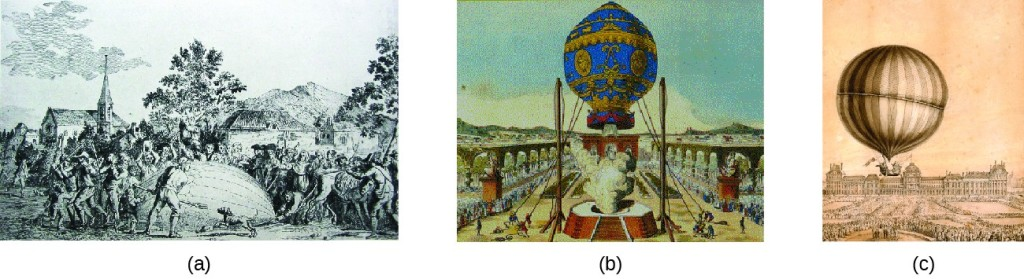Figure 1. In 1783, the first (a) hydrogen-filled balloon flight, (b) manned hot air balloon flight, and (c) manned hydrogen-filled balloon flight occurred. When the hydrogen-filled balloon depicted in (a) landed, the frightened villagers of Gonesse reportedly destroyed it with pitchforks and knives. The launch of the latter was reportedly viewed by 400,000 people in Paris.

## Pressure and Temperature: Gay-Lussac’s Law

Imagine filling a rigid container attached to a pressure gauge with gas and then sealing the container so that no gas may escape. If the container is cooled, the gas inside likewise gets colder and its pressure is observed to decrease. Since the container is rigid and tightly sealed, both the volume and number of moles of gas remain constant. If we heat the sphere, the gas inside gets hotter (Figure 2) and the pressure increases.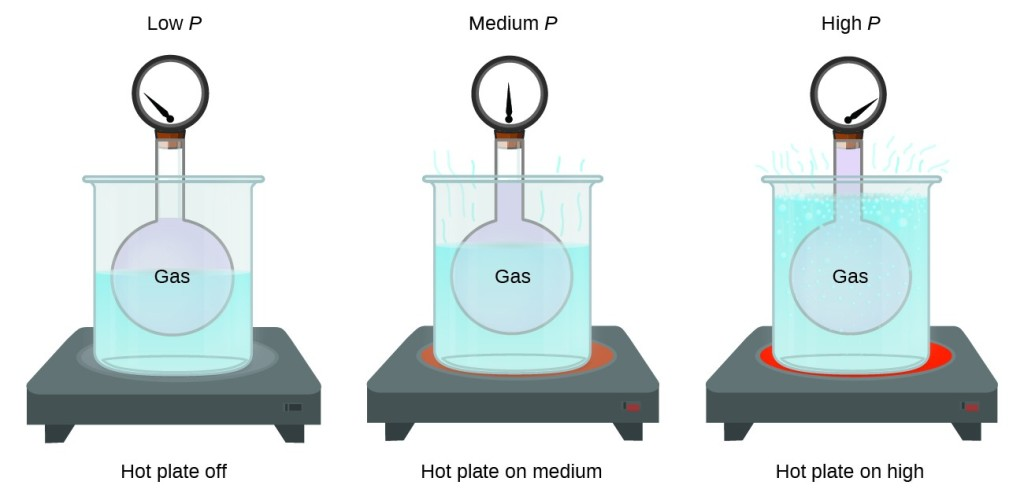Figure 2. The effect of temperature on gas pressure: When the hot plate is off, the pressure of the gas in the sphere is relatively low. As the gas is heated, the pressure of the gas in the sphere increases.

This relationship between temperature and pressure is observed for any sample of gas confined to a constant volume. An example of experimental pressure-temperature data is shown for a sample of air under these conditions in Figure 3. We find that temperature and pressure are linearly related, and if the temperature is on the kelvin scale, then P and T are directly proportional (again, when volume and moles of gas are held constant); if the temperature on the kelvin scale increases by a certain factor, the gas pressure increases by the same factor.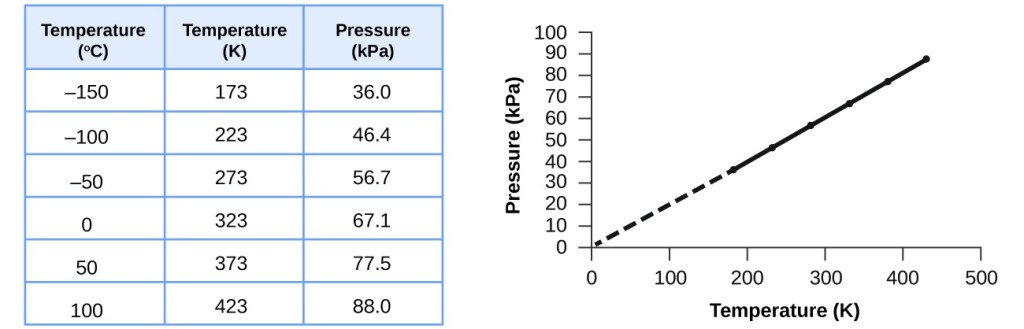Figure 3. For a constant volume and amount of air, the pressure and temperature are directly proportional, provided the temperature is in kelvin. (Measurements cannot be made at lower temperatures because of the condensation of the gas.) When this line is extrapolated to lower pressures, it reaches a pressure of 0 at –273 °C, which is 0 on the kelvin scale and the lowest possible temperature, called absolute zero.

Guillaume Amontons was the first to empirically establish the relationship between the pressure and the temperature of a gas (~1700), and Joseph Louis Gay-Lussac determined the relationship more precisely (~1800). Because of this, the PT relationship for gases is known as either Gay-Lussac’s law or Amontons’s Law. Under either name, it states that the pressure of a given amount of gas is directly proportional to its temperature on the kelvin scale when the volume is held constant. Mathematically, this can be written:

$\large P\propto T$

$\large P=\text{constant}\times T$

$\large P=k\times T$

where ∝ means “is proportional to,” and k is a proportionality constant that depends on the identity, amount, and volume of the gas.

For a confined, constant volume of gas, the ratio $\large\frac{P}{T}$ is therefore constant (i.e., $\large\frac{P}{T}=k$ ). If the gas is initially in “Condition 1” (with P = P1 and T = T1), and then changes to “Condition 2” (with P = P2 and T = T2), we have that $\large\frac{{P}_{1}}{{T}_{1}}=k$ and $\frac{{P}_{2}}{{T}_{2}}=k,$ which reduces to:

$\large\frac{{P}_{1}}{{T}_{1}}=\frac{{P}_{2}}{{T}_{2}}$

This equation is useful for pressure-temperature calculations for a confined gas at constant volume. Note that temperatures must be on the kelvin scale for any gas law calculations (0 on the kelvin scale and the lowest possible temperature is called absolute zero). (Also note that there are at least three ways we can describe how the pressure of a gas changes as its temperature changes: We can use a table of values, a graph, or a mathematical equation.)

### Example 1: Predicting Change in Pressure with Temperature

A can of hair spray is used until it is empty except for the propellant, isobutane gas.

1. On the can is the warning “Store only at temperatures below 120 °F (48.8 °C). Do not incinerate.” Why?
2. The gas in the can is initially at 24 °C and 360 kPa, and the can has a volume of 350 mL. If the can is left in a car that reaches 50 °C on a hot day, what is the new pressure in the can?

A sample of nitrogen, N2, occupies 45.0 mL at 27 °C and 600 torr. What pressure will it have if cooled to –73 °C while the volume remains constant?

## Volume and Temperature: Charles’s Law

If we fill a balloon with air and seal it, the balloon contains a specific amount of air at atmospheric pressure, let’s say 1 atm. If we put the balloon in a refrigerator, the gas inside gets cold and the balloon shrinks (although both the amount of gas and its pressure remain constant). If we make the balloon very cold, it will shrink a great deal, and it expands again when it warms up.

This video shows how cooling and heating a gas causes its volume to decrease or increase, respectively.

These examples of the effect of temperature on the volume of a given amount of a confined gas at constant pressure are true in general: The volume increases as the temperature increases, and decreases as the temperature decreases. Volume-temperature data for a 1-mole sample of methane gas at 1 atm are listed and graphed in Figure 4.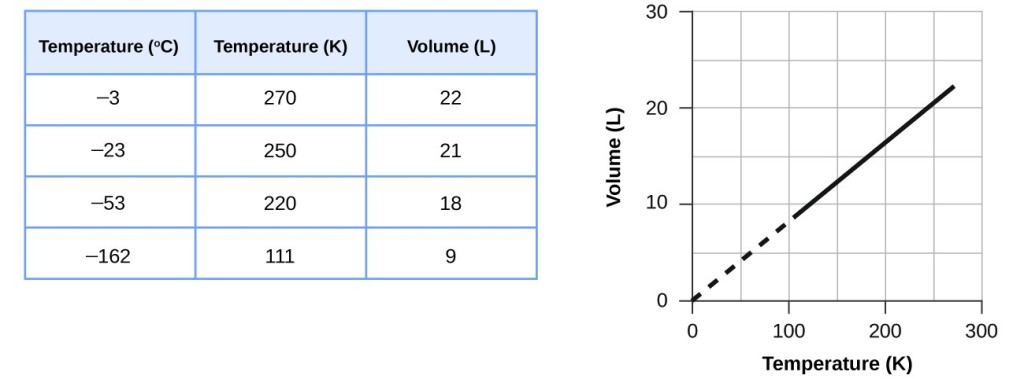Figure 4. The volume and temperature are linearly related for 1 mole of methane gas at a constant pressure of 1 atm. If the temperature is in kelvin, volume and temperature are directly proportional. The line stops at 111 K because methane liquefies at this temperature; when extrapolated, it intersects the graph’s origin, representing a temperature of absolute zero.

The relationship between the volume and temperature of a given amount of gas at constant pressure is known as Charles’s law in recognition of the French scientist and balloon flight pioneer Jacques Alexandre César Charles. Charles’s law states that the volume of a given amount of gas is directly proportional to its temperature on the kelvin scale when the pressure is held constant.

Mathematically, this can be written as:

$\large V\propto T$

$\large V=\text{constant}\cdot T$

$\large V=k\cdot T$

with k being a proportionality constant that depends on the amount and pressure of the gas.

For a confined, constant pressure gas sample, $\large\frac{V}{T}$ is constant (i.e., the ratio = k), and as seen with the VT relationship, this leads to another form of Charles’s law:

$\large\frac{{V}_{1}}{{T}_{1}}=\frac{{V}_{2}}{{T}_{2}}$

### Example 2: Predicting Change in Volume with Temperature

A sample of carbon dioxide, CO2, occupies 0.300 L at 10 °C and 750 torr. What volume will the gas have at 30 °C and 750 torr?

A sample of oxygen, O2, occupies 32.2 mL at 30 °C and 452 torr. What volume will it occupy at –70 °C and the same pressure?

### Example 3: Measuring Temperature with a Volume Change

Temperature is sometimes measured with a gas thermometer by observing the change in the volume of the gas as the temperature changes at constant pressure. The hydrogen in a particular hydrogen gas thermometer has a volume of 150.0 cm3 when immersed in a mixture of ice and water (0.00 °C). When immersed in boiling liquid ammonia, the volume of the hydrogen, at the same pressure, is 131.7 cm3. Find the temperature of boiling ammonia on the kelvin and Celsius scales.

What is the volume of a sample of ethane at 467 K and 1.1 atm if it occupies 405 mL at 298 K and 1.1 atm?

## Volume and Pressure: Boyle’s Law

If we partially fill an airtight syringe with air, the syringe contains a specific amount of air at constant temperature, say 25 °C. If we slowly push in the plunger while keeping temperature constant, the gas in the syringe is compressed into a smaller volume and its pressure increases; if we pull out the plunger, the volume increases and the pressure decreases. This example of the effect of volume on the pressure of a given amount of a confined gas is true in general. Decreasing the volume of a contained gas will increase its pressure, and increasing its volume will decrease its pressure. In fact, if the volume increases by a certain factor, the pressure decreases by the same factor, and vice versa. Volume-pressure data for an air sample at room temperature are graphed in Figure 5.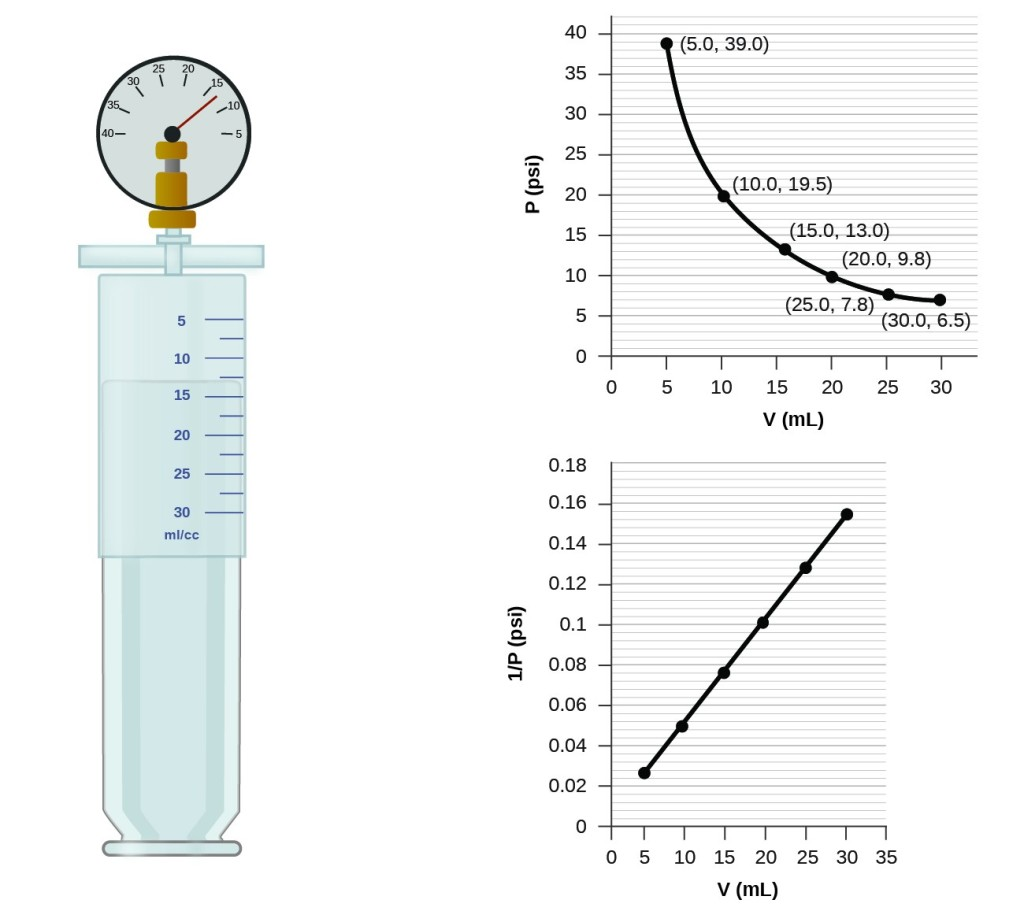Figure 5. When a gas occupies a smaller volume, it exerts a higher pressure; when it occupies a larger volume, it exerts a lower pressure (assuming the amount of gas and the temperature do not change). Since P and V are inversely proportional, a graph of 1/P vs. V is linear.

Unlike the PT and VT relationships, pressure and volume are not directly proportional to each other. Instead, P and V exhibit inverse proportionality: Increasing the pressure results in a decrease of the volume of the gas. Mathematically this can be written:

$\large P\propto 1\text{/}V\text{ or }P=k\cdot 1\text{/}V\text{ or }P\cdot V=k\text{ or }{P}_{1}{V}_{1}={P}_{2}{V}_{2}$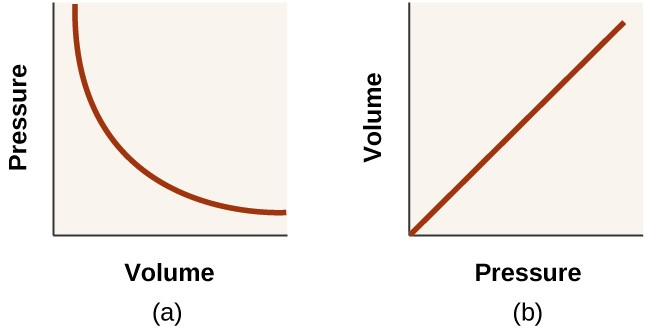Figure 6. The relationship between pressure and volume is inversely proportional. (a) The graph of P vs. V is a parabola, whereas (b) the graph of (1/P) vs. V is linear.

with k being a constant. Graphically, this relationship is shown by the straight line that results when plotting the inverse of the pressure $\large\left(\frac{1}{P}\right)$ versus the volume (V), or the inverse of volume $\large\left(\frac{1}{V}\right)$ versus the pressure (V). Graphs with curved lines are difficult to read accurately at low or high values of the variables, and they are more difficult to use in fitting theoretical equations and parameters to experimental data. For those reasons, scientists often try to find a way to “linearize” their data. If we plot P versus V, we obtain a hyperbola (see Figure 6).

The relationship between the volume and pressure of a given amount of gas at constant temperature was first published by the English natural philosopher Robert Boyle over 300 years ago. It is summarized in the statement now known as Boyle’s law: The volume of a given amount of gas held at constant temperature is inversely proportional to the pressure under which it is measured.

### Example 4: Volume of a Gas Sample

The sample of gas in Figure 5 has a volume of 15.0 mL at a pressure of 13.0 psi. Determine the pressure of the gas at a volume of 7.5 mL, using:

1. the PV graph in Figure 5
2. the $\large\frac{1}{P}$ vs. V graph in Figure 5
3. the Boyle’s law equation

Comment on the likely accuracy of each method.

The sample of gas in Figure 5 has a volume of 30.0 mL at a pressure of 6.5 psi. Determine the volume of the gas at a pressure of 11.0 mL, using:

1. the PV graph in Figure 5
2. the $\frac{1}{P}$ vs. V graph in Figure 5
3. the Boyle’s law equation

Comment on the likely accuracy of each method.

### Chemistry in Action: Breathing and Boyle’s Law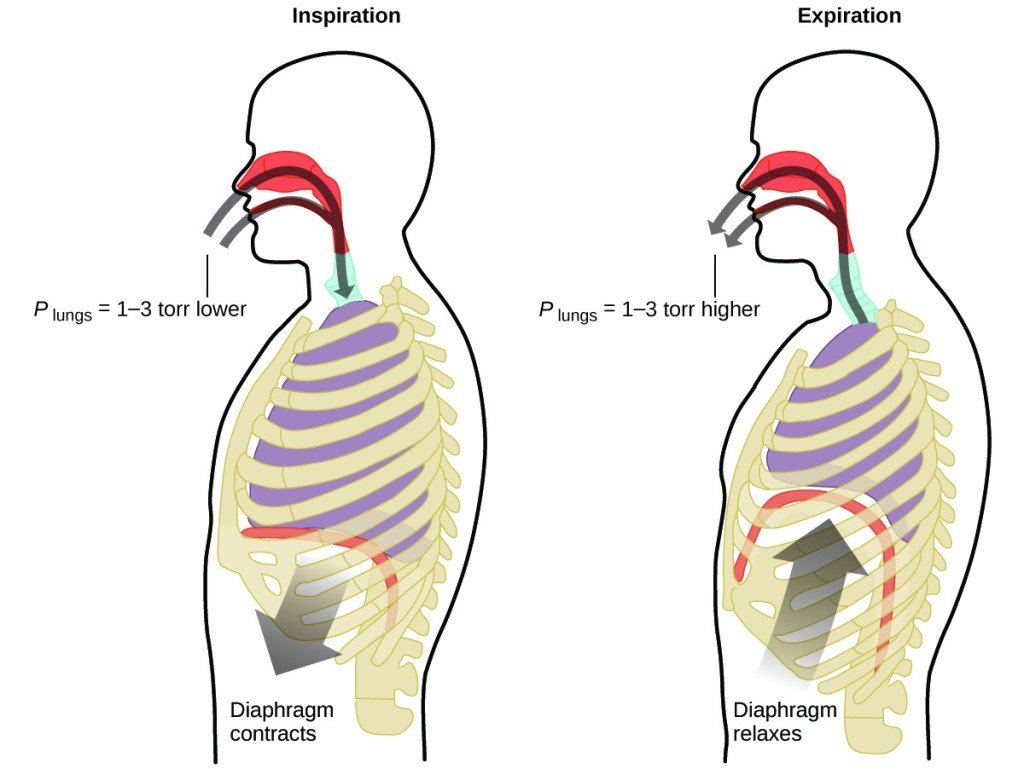Figure 7. Breathing occurs because expanding and contracting lung volume creates small pressure differences between your lungs and your surroundings, causing air to be drawn into and forced out of your lungs.

## Moles of Gas and Volume: Avogadro’s Law

The Italian scientist Amedeo Avogadro advanced a hypothesis in 1811 to account for the behavior of gases, stating that equal volumes of all gases, measured under the same conditions of temperature and pressure, contain the same number of molecules. Over time, this relationship was supported by many experimental observations as expressed by Avogadro’s law: For a confined gas, the volume (V) and number of moles (n) are directly proportional if the pressure and temperature both remain constant.

In equation form, this is written as:

$\large\begin{array}{ccccc}V\propto n& \text{or}& V=k\times n& \text{or}& \frac{{V}_{1}}{{n}_{1}}=\frac{{V}_{2}}{{n}_{2}}\end{array}$

Mathematical relationships can also be determined for the other variable pairs, such as P versus n, and n versus T.

Visit this interactive PhET simulation link to investigate the relationships between pressure, volume, temperature. and amount of gas. Use the simulation to examine the effect of changing one parameter on another while holding the other parameters constant (as described in the preceding sections on the various gas laws).

### Example 5: Measuring Volume with Change in moles

A 2.45 L volume of gas contains 4.5 moles of gas. How many moles of gas are there in 3.87 L if the gas is at constant pressure and temperature?

A 12.8 L volume of gas contains 3.00 moles of gas. At constant temperature and pressure, what will be the volume of gas if 5.22 moles of gas is added?

## The Combined Gas Law

One thing we notice about all the gas laws is that, collectively, volume and pressure are always in the numerator, and temperature is always in the denominator. This suggests that we can propose a gas law that combines pressure, volume, and temperature. This gas law is known as the combined gas law, and its mathematical form is

$\large\frac{{P}_{1}{V}_{1}}{{T}_{1}}=\frac{{P}_{2}{V}_{2}}{{T}_{2}}\text{ with n constant}$

This allows us to follow changes in all three major properties of a gas. Again, the usual warnings apply about how to solve for an unknown algebraically (isolate it on one side of the equation in the numerator), units (they must be the same for the two similar variables of each type), and units of temperature (must be in kelvins).

### Example 6: Combined gas law

A sample of gas at an initial volume of 8.33 L, an initial pressure of 1.82 atm, and an initial temperature of 286 K simultaneously changes its temperature to 355 K and its volume to 5.72 L. What is the final pressure of the gas?

A sample of gas at an initial volume of 46.7 mL, an initial pressure of 662 torr, and an initial temperature of 266 K simultaneously changes its temperature to 371 K and its pressure to 40 torr. What is the final volume of the gas?

As with other gas laws, if you need to determine the value of a variable in the denominator of the combined gas law, you can either cross-multiply all the terms or just take the reciprocal of the combined gas law. Remember, the variable you are solving for must be in the numerator and all by itself on one side of the equation.

### Key Concepts and Summary

The behavior of gases can be described by several laws based on experimental observations of their properties. The pressure of a given amount of gas is directly proportional to its absolute temperature, provided that the volume does not change (Gay-Lussac’s law). The volume of a given amount of gas sample is directly proportional to its absolute temperature at constant pressure (Charles’s law). The volume of a given amount of gas is inversely proportional to its pressure when temperature is held constant (Boyle’s law). Under the same conditions of temperature and pressure, equal volumes of all gases contain the same number of molecules (Avogadro’s law).

#### Key Equations

• Gay-Lussac’s Law: $\large\frac{{P}_{1}}{{T}_{1}}=\frac{{P}_{2}}{{T}_{2}}$
• Charles’s Law: $\large\frac{{V}_{1}}{{T}_{1}}=\frac{{V}_{2}}{{T}_{2}}$
• Boyle’s Law: $\large{P}_{1}{V}_{1}={P}_{2}{V}_{2}$
• Combined Gas Law: $\large\frac{{P}_{1}{V}_{1}}{{T}_{1}}=\frac{{P}_{2}{V}_{2}}{{T}_{2}}\text{ with n constant}$

### Exercises

1. Sometimes leaving a bicycle in the sun on a hot day will cause a blowout. Why?
2. Explain how the volume of the bubbles exhausted by a scuba diver (Figure 8) change as they rise to the surface, assuming that they remain intact.
3. One way to state Boyle’s law is “All other things being equal, the pressure of a gas is inversely proportional to its volume.”
1. What is the meaning of the term “inversely proportional?”
2. What are the “other things” that must be equal?
4. An alternate way to state Avogadro’s law is “All other things being equal, the number of molecules in a gas is directly proportional to the volume of the gas.”
1. What is the meaning of the term “directly proportional?”
2. What are the “other things” that must be equal?
5. How would the graph in Figure 4 change if the number of moles of gas in the sample used to determine the curve were doubled?
6. How would the graph in Figure 5 change if the number of moles of gas in the sample used to determine the curve were doubled?
7. In addition to the data found in Figure 5, what other information do we need to find the mass of the sample of air used to determine the graph?
8. Determine the volume of 1 mol of CH4 gas at 150 K and 1 atm, using Figure 4.
9. Determine the pressure of the gas in the syringe shown in Figure 5 when its volume is 12.5 mL, using:
1. the appropriate graph
2. Boyle’s law
10. A spray can is used until it is empty except for the propellant gas, which has a pressure of 1344 torr at 23 °C. If the can is thrown into a fire (T = 475 °C), what will be the pressure in the hot can?
11. What is the temperature of an 11.2 L sample of carbon monoxide, CO, at 744 torr if it occupies 13.3 L at 55 °C and 744 torr?
12. A 2.50 L volume of hydrogen measured at –196 °C is warmed to 100 °C. Calculate the volume of the gas at the higher temperature, assuming no change in pressure.
13. A balloon inflated with three breaths of air has a volume of 1.7 L. At the same temperature and pressure, what is the volume of the balloon if five more same-sized breaths are added to the balloon?
14. A weather balloon contains 8.80 moles of helium at a pressure of 0.992 atm and a temperature of 25 °C at ground level. What is the volume of the balloon under these conditions?
15. The volume of an automobile air bag was 66.8 L when inflated at 25 °C with 77.8 g of nitrogen gas. What was the pressure in the bag in kPa?
16. How many moles of gaseous boron trifluoride, BF3, are contained in a 4.3410 L bulb at 788.0 K if the pressure is 1.220 atm? How many grams of BF3?
17. Iodine, I2, is a solid at room temperature but sublimes (converts from a solid into a gas) when warmed. What is the temperature in a 73.3 mL bulb that contains 0.292 g of I2 vapor at a pressure of 0.462 atm?
18. How many grams of gas are present in each of the following cases?
1. 0.100 L of CO2 at 307 torr and 26 °C
2. 8.75 L of C2H4, at 378.3 kPa and 483 K
3. 221 mL of Ar at 0.23 torr and –54 °C
19. A high altitude balloon is filled with 1.41 × 104 L of hydrogen at a temperature of 21 °C and a pressure of 745 torr. What is the volume of the balloon at a height of 20 km, where the temperature is –48 °C and the pressure is 63.1 torr?
20. A cylinder of medical oxygen has a volume of 35.4 L, and contains O2 at a pressure of 151 atm and a temperature of 25 °C. What volume of O2 does this correspond to at normal body conditions, that is, 1 atm and 37 °C?
21. A large scuba tank (Figure 8) with a volume of 18 L is rated for a pressure of 220 bar. The tank is filled at 20 °C and contains enough air to supply 1860 L of air to a diver at a pressure of 2.37 atm (a depth of 45 feet). Was the tank filled to capacity at 20 °C?
22. A 20.0 L cylinder containing 11.34 kg of butane, C4H10, was opened to the atmosphere. Calculate the mass of the gas remaining in the cylinder if it were opened and the gas escaped until the pressure in the cylinder was equal to the atmospheric pressure, 0.983 atm, and a temperature of 27 °C.
23. While resting, the average 70 kg human male consumes 14 L of pure O2 per hour at 25 °C and 100 kPa. How many moles of O2 are consumed by a 70 kg man while resting for 1.0 h?
24. For a given amount of gas showing ideal behavior, draw labeled graphs of:
1. the variation of P with V
2. the variation of V with T
3. the variation of P with T
4. the variation of $\frac{1}{P}$ with V
25. A liter of methane gas, CH4, at STP contains more atoms of hydrogen than does a liter of pure hydrogen gas, H2, at STP. Using Avogadro’s law as a starting point, explain why.
26. The effect of chlorofluorocarbons (such as CCl2F2) on the depletion of the ozone layer is well known. The use of substitutes, such as CH3CH2F(g), for the chlorofluorocarbons, has largely corrected the problem. Calculate the volume occupied by 10.0 g of each of these compounds at STP:
1. CCl2F2(g)
2. CH3CH2F(g)
27. As 1 g of the radioactive element radium decays over 1 year, it produces 1.16 × 1018 alpha particles (helium nuclei). Each alpha particle becomes an atom of helium gas. What is the pressure in pascal of the helium gas produced if it occupies a volume of 125 mL at a temperature of 25 °C?
28. A balloon that is 100.21 L at 21 °C and 0.981 atm is released and just barely clears the top of Mount Crumpet in British Columbia. If the final volume of the balloon is 144.53 L at a temperature of 5.24 °C, what is the pressure experienced by the balloon as it clears Mount Crumpet?
29. If the temperature of a fixed amount of a gas is doubled at constant volume, what happens to the pressure?
30. If the volume of a fixed amount of a gas is tripled at constant temperature, what happens to the pressure?
31. A gas starts at the conditions 78.9 mL, 3.008 atm, and 56°C. Its conditions change to 35.6 mL and 2.55 atm. What is its final temperature?

32. The initial conditions of a sample of gas are 319 K, 3.087 L, and 591 torr. What is its final pressure if volume is changed to 2.222 L and temperature is changed to 299 K?

33. A gas starts with initial pressure of 7.11 atm, initial temperature of 66°C, and initial volume of 90.7 mL. If its conditions change to 33°C and 14.33 atm, what is its final volume?

## Glossary

absolute zero: temperature at which the volume of a gas would be zero according to Charles’s law.

Avogadro’s law: volume of a gas at constant temperature and pressure is proportional to the number of gas molecules

Boyle’s law: volume of a given number of moles of gas held at constant temperature is inversely proportional to the pressure under which it is measured

Charles’s law: volume of a given number of moles of gas is directly proportional to its kelvin temperature when the pressure is held constant

Gay-Lussac’s law: (also, Amontons’s law) pressure of a given number of moles of gas is directly proportional to its kelvin temperature when the volume is held constant